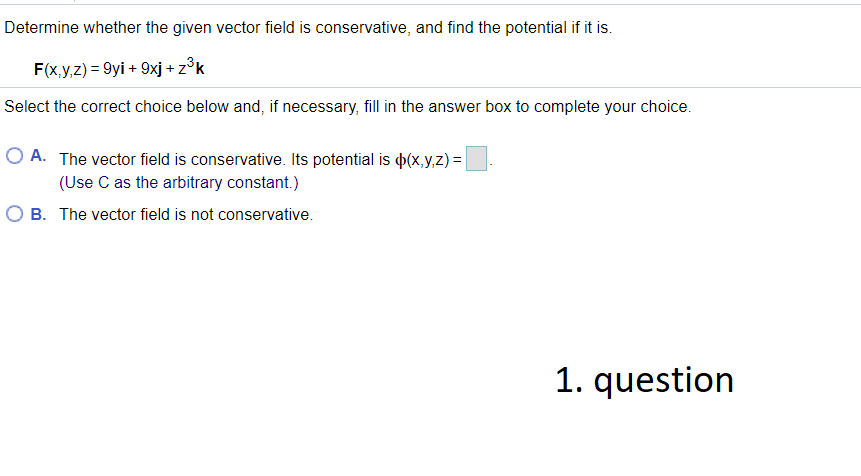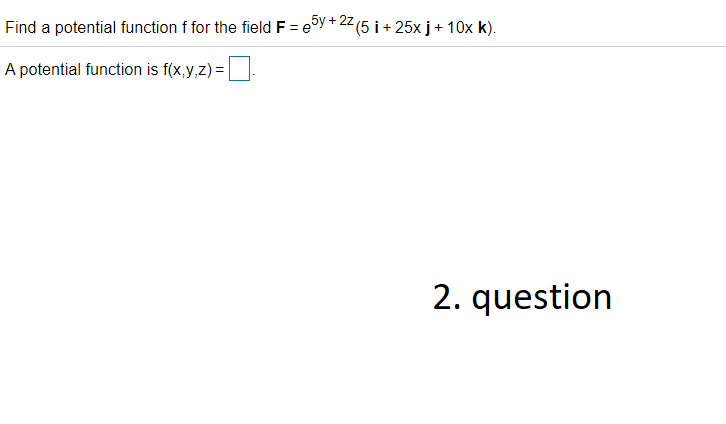### Create an Account

Already have account?

### Forgot Your Password ?

Home / Questions / Determine whether the given vector field is conservative, and find the potential if it is....

# Determine whether the given vector field is conservative, and find the potential if it is. F(x,y,z) = 9yi + 9xj + zºk Select the correct choice below and, if necessary, fill in the answer box to compl

Determine whether the given vector field is conservative, and find the potential if it is. F(x,y,z) = 9yi + 9xj + zºk Select the correct choice below and, if necessary, fill in the answer box to complete your choice. O A. The vector field is conservative. Its potential is \$(x,y,z) = (Use C as the arbitrary constant.) O B. The vector field is not conservative. 1. question
Find a potential function f for the field F = 25y +22(5 i + 25x j + 10x K). A potential function is f(x,y,z) = 2. questionCould you please solve these two questions?

Apr 29 2021 View more View Less

#### Answer (Solved)Subscribe To Get Solution